# MA.8.AR.1.1Export Print
Apply the Laws of Exponents to generate equivalent algebraic expressions, limited to integer exponents and monomial bases.

### Examples

The expression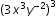is equivalent to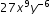.

### Clarifications

Clarification 1: Refer to the K-12 Formulas (Appendix E) for the Laws of Exponents.
General Information
Subject Area: Mathematics (B.E.S.T.)
Strand: Algebraic Reasoning
Status: State Board Approved

## Benchmark Instructional Guide

• Base
• Expression
• Integers
• Monomial

### Vertical Alignment

Previous Benchmarks

Next Benchmarks

### Purpose and Instructional Strategies

In Grade 7, students applied the Laws of Exponents to evaluate and generate numerical expressions, limited to whole-number exponents and rational number bases. In Grade 8, students extend their knowledge of the Laws of Exponents to generate equivalent algebraic expressions with integer exponents and monomial bases. In Algebra 1, students will use their knowledge of the Laws of Exponents to generate equivalent algebraic expressions with rational and variable exponents.
• At the onset of learning about exponents, students learn that it is a way to write expanded multiplication in a more condensed form. The understanding that the number which is referred to as the base is multiplied times itself based on the value of the exponent is foundational.
• This benchmark can be paired with MA.8.NSO.1.3 which helps students work within numerical expressions with integer exponents and rational bases. Students should move from numerical expressions to algebraic expressions to best enhance their conceptual understanding of the Laws of Exponents.
• A strategy for developing meaning for integer exponents is to make use of patterns as shown below: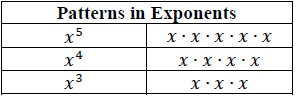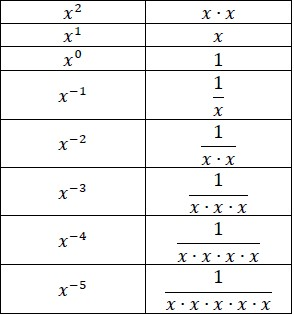• Teach one law at a time to allow for conceptual understanding instead of memorizing the rules. Students should not be told the properties but rather should derive them through experience and reason. During instruction, include examples that show the expansion of the bases with the use of the exponents to show equivalence.
• For mastery of this benchmark, monomials can be defined in the following way: a base may be a product of a coefficient and one or more variables with integer exponents. This limitation should not prevent students from understanding that a negative exponent can be represented equivalently as a positive exponent with the reciprocal base (changing numerator to denominator or denominator to numerator).

### Common Misconceptions or Errors

• When working with negative exponents, students may not understand the connection to fractions and values in the denominator.
• Students incorrectly multiply the exponent with the base number.
• Students may incorrectly apply the Laws of Exponents.

### Strategies to Support Tiered Instruction

• Teachers should review exponents as condensed multiplication and write out expanded form, and provide opportunities to notice patterns as discussed in MA.8.NSO.1.3. Teachers can use the “Patterns in Exponents” chart shown in the Purpose and Instructional Strategies section with the right-side blank so that students can begin to complete and understand the patterns of exponents.
• Teachers should re-emphasize the structure of exponents, and how they are used by multiplying the base by itself the number of times as notated by the exponent.
• Teacher provides a review of the relationship between the base and the exponent by modeling an example of operations using a base and exponent.
• For example, determine the numerical value of 6³.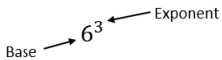6³ which is equivalent to 6⋅6⋅6 which is equivalent to 216.

Two students were working on generating equivalent expressions for (15$x$$y$²)³, and showed their solutions below.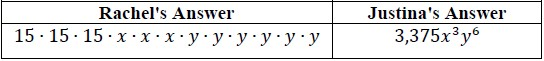The teacher said Rachel and Justina both have the correct answer. Do you agree with the teacher? Explain your reasoning.

Create a pattern using the expanded form of the base, $x$, between $x$−5 and $x$5. Explain why $x$0 is equal to 1.

### Instructional Items

Instructional Item 1
Write $x$5$x$8 with the variable $x$ used only one time.

Instructional Item 2
An expression is given.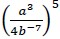Write an equivalent expression with only two exponents and no negative exponents.

Instructional Item 3
Write $y$−3$z$−4 with only positive exponents.

*The strategies, tasks and items included in the B1G-M are examples and should not be considered comprehensive.

## Related Courses

This benchmark is part of these courses.
1205050: M/J Accelerated Mathematics Grade 7 (Specifically in versions: 2014 - 2015, 2015 - 2020, 2020 - 2022, 2022 and beyond (current))
1205070: M/J Grade 8 Pre-Algebra (Specifically in versions: 2014 - 2015, 2015 - 2022, 2022 and beyond (current))
1204000: M/J Foundational Skills in Mathematics 6-8 (Specifically in versions: 2014 - 2015, 2015 - 2022, 2022 and beyond (current))
7812030: Access M/J Grade 8 Pre-Algebra (Specifically in versions: 2014 - 2015, 2015 - 2018, 2018 - 2019, 2019 - 2022, 2022 and beyond (current))

## Related Access Points

Alternate version of this benchmark for students with significant cognitive disabilities.
MA.8.AR.1.AP.1: Use the properties of integer exponents and product/quotient of powers with like bases to produce equivalent algebraic expressions limited to positive exponents and monomial bases.

## Related Resources

Vetted resources educators can use to teach the concepts and skills in this benchmark.

## Tutorial

Exponents with Negative Bases:

In this tutorial, you will apply what you know about multiplying negative numbers to determine how negative bases with exponents are affected and what patterns develop.

Type: Tutorial

## Student Resources

Vetted resources students can use to learn the concepts and skills in this benchmark.

## Tutorial

Exponents with Negative Bases:

In this tutorial, you will apply what you know about multiplying negative numbers to determine how negative bases with exponents are affected and what patterns develop.

Type: Tutorial

## Parent Resources

Vetted resources caregivers can use to help students learn the concepts and skills in this benchmark.